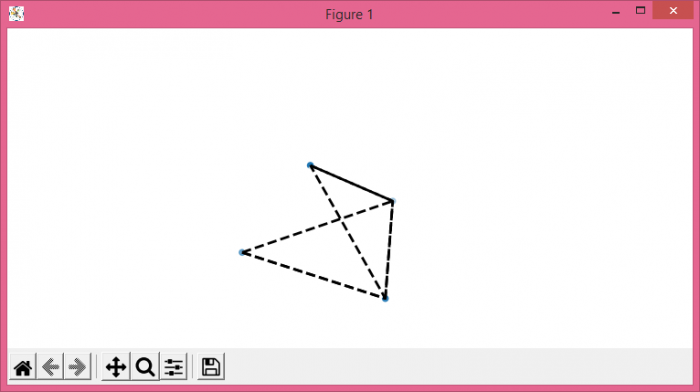# Transparency for Poly3DCollection plot in Matplotlib

To plot a transparent Poly3DCollection plot in Matplotlib, we can take the following steps −

• Set the figure size and adjust the padding between and around the subplots
• Create a new figure or activate an existing figure.
• Add an '~.axes.Axes' to the figure as part of a subplot arrangement with projection=3d.
• Create x, y and z data points.
• Make a list of vertices.
• Convert x, y and z data points into a zipped list of tuples.
• Get a list of instance of Poly3d.
• Add a 3D collection object to the plot using add_collection3d() method.
• Turn off the axes.
• To display the figure, use show() method.

## Example

from matplotlib import pyplot as plt
from mpl_toolkits.mplot3d.art3d import Poly3DCollection, Line3DCollection

plt.rcParams["figure.figsize"] = [7.50, 3.50]
plt.rcParams["figure.autolayout"] = True

fig = plt.figure()

x = [0, 2, 1, 1]
y = [0, 0, 1, 0]
z = [0, 0, 0, 1]

vertices = [[0, 1, 2], [0, 1, 3], [0, 2, 3], [1, 2, 3]]
tupleList = list(zip(x, y, z))
poly3d = [[tupleList[vertices[ix][iy]]
for iy in range(len(vertices))]
for ix in range(len(vertices))]

ax.scatter(x, y, z)
plt.show()